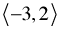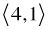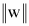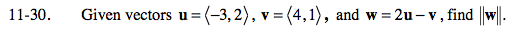Home > PC > Chapter 11 > Lesson 11.1.2 > Problem11-30

11-30.

Given vectors u =, v =, and w = 2u − v, find. Homework Help ✎$\bold\text{w} = 2\left\langle -3,2 \right\rangle -\left\langle 4,1 \right\rangle$

Once you find vector w, sketch it and use the Pythagorean theorem to find its length, or ||w||.